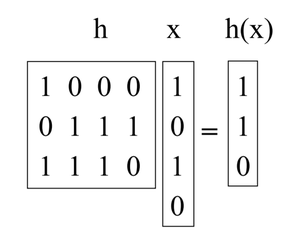GFG App
Open AppBrowser
Continue

# Introduction to Universal Hashing in Data Structure

Universal hashing is a technique used in computer science and information theory for designing hash functions. It is a family of hash functions that can be efficiently computed by using a randomly selected hash function from a set of hash functions. The goal of universal hashing is to minimize the chance of collisions between distinct keys, which can lead to degraded performance in hash table operations.

In the traditional approach to hashing, a fixed hash function is used to map keys to an array index. However, if the distribution of keys is not uniform, collisions may occur frequently, causing the hash table to degrade into a linked list, which can severely impact performance.

Universal hashing attempts to solve this problem by choosing a hash function at random from a family of hash functions. The family of hash functions is designed to minimize the probability of collisions, regardless of the distribution of keys. By randomly selecting a hash function from the family for each new key, the chance of collisions is further reduced.

### Universal hashing has several advantages, including:

It provides a high degree of randomness in the selection of hash functions, which reduces the likelihood of collisions.
It is simple to implement and can be used in a wide variety of applications.
It has a high degree of flexibility, allowing for easy modification of the family of hash functions to optimize performance for specific data sets.

### However, there are also some disadvantages to using universal hashing, including:

It can be computationally expensive to generate a large number of hash functions.
The selection of hash functions must be done carefully to ensure they are truly independent.
It may not be suitable for all types of data sets, particularly those with complex or unpredictable distributions.
Some recommended books on universal hashing and hash tables include “Algorithms” by Robert Sedgewick and Kevin Wayne, “Introduction to Algorithms” by Thomas H. Cormen, Charles E. Leiserson, Ronald L. Rivest, and Clifford Stein, and “The Art of Computer Programming” by Donald E. Knuth.

Hashing is a great practical tool, with an interesting and subtle theory too. In addition to its use as a dictionary data structure, hashing also comes up in many different areas, including cryptography and complexity theory

Universal Hashing refers to selecting a hash function at random from a family of hash functions with a certain mathematical property. This ensures a minimum number of collisions.

A randomized algorithm H for constructing hash functions h : U → {1,… ,M} is universal if for all (x, y) in U such that x ≠ y, Pr h∈H [h(x) = h(y)] ≤ 1/M (i.e, The probability of x and y such that h(x) = h(y) is <= 1/M for all possible values of x and y).

A set H of hash functions is called a universal hash function family if the procedure choose h ∈ H at random is universal. (Here the key is identifying the set of functions with the uniform distribution over the set.)

Theorem: If H is a set of the universal hash function family, then for any set S ⊆ U of size N, such that x ∈ U and y ∈ S, the expected number of collisions between x and y is at most N/M.

Proof: Each y ∈ S (y ≠ x) has at most a 1/M chance of colliding with x by the definition of “universal”. So,

• Let Cxy = 1 if x and y collide and 0 otherwise.
• Let Cx denote the total number of collisions for x. So, Cx = ∑ y∈S, x≠y Cxy.
• We know E[Cxy] = Pr[ x and y collide ] ≤ 1/M.
• So, by the linearity of expectation, E[Cx] = ∑y E[Cxy] < N/M.

Corollary: If H is a set of the universal hash function family, then for any sequence of L insert, lookup, or delete operations in which there are at most M elements in the system at any time, the expected total cost of the L operations for a random h ∈ H is only O(L) (viewing the time to compute h as constant).

For any given operation in the sequence, its expected cost is constant by the above theorem. Therefore, the expected total cost of the L operations is O(L) by the linearity of expectation.

Constructing a universal hash family using the matrix method:

Let’s say keys are u-bits long and the table size M is the power of 2, so an index is b-bits long with M = 2b.

What we will do is pick h to be a random b-by-u binary matrix, and define h(x) = hx, where hx is calculated by adding some of the columns of h (doing vector addition over mod 2) where the 1 bits in x indicate which columns to add. (e.g., the 1st and 3rd columns of h are added in the below example). These matrices are short and fat. For instance:Now, take an arbitrary pair of keys (x, y) such that x ≠ y. They must differ someplace, let’s assume they differ in the ith coordinate, and for concreteness say xi = 0 and yi = 1. Imagine we first choose all of h but the ith column. Over the remaining choices of the ith column, h(x) is fixed. However, each of the 2b different settings of the ith column gives a different value of h(y) (in particular, every time we flip a bit in that column, we flip the corresponding bit in h(y)). So there is exactly a 1/2b chance that h(x) = h(y).

My Personal Notes arrow_drop_up